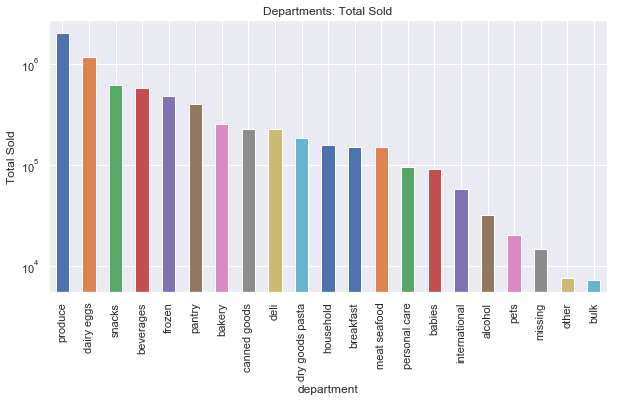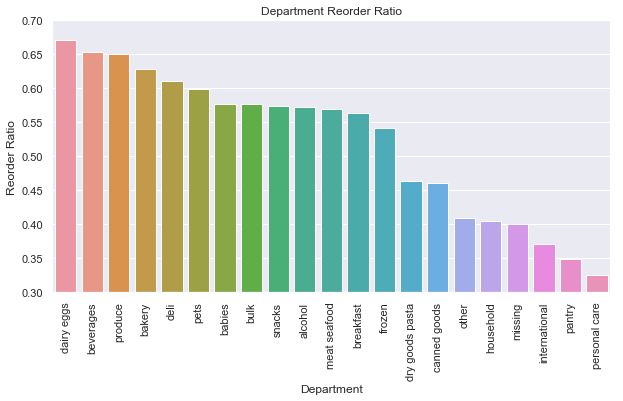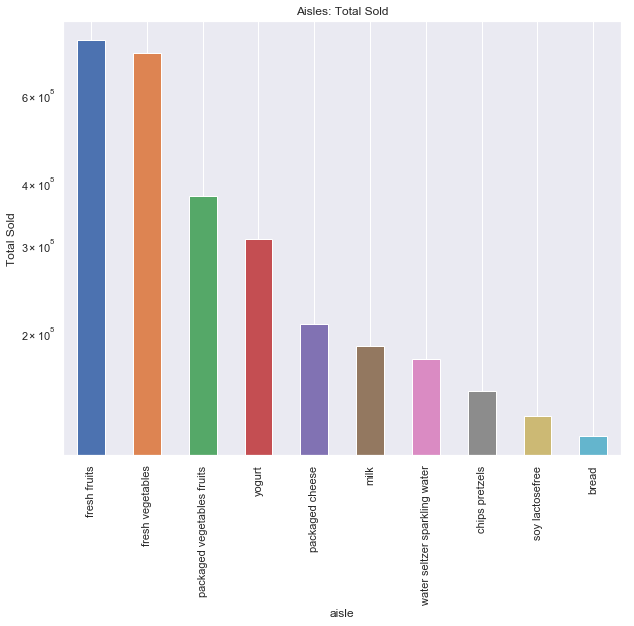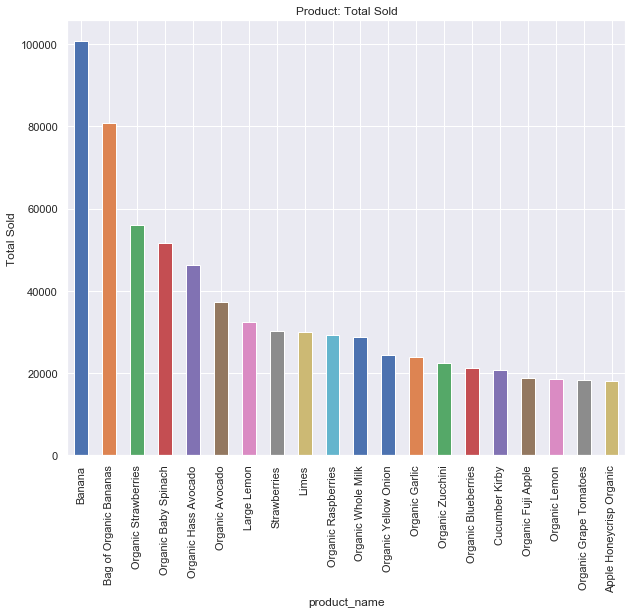# Data Story, Instacart #3

For my nest efforts, I am working in Python and MySQL. MySQL might be a little light weight for this quantity of data, but here are the results so far:

### Setting up the database

For this analysis, I have loaded the data files into a MySQL database. I chose MySQL because I wanted to be able to build on any data exploration I conducted and make it available to other platforms such as Tableau.

In :
```import time
import mysql.connector

import pandas as pd
#import numpy as np
import seaborn as sns
import matplotlib.pyplot as plt

from insta_config import (USR, PASS, HST, DB)

%matplotlib inline

sns.set(color_codes=True)
color = sns.color_palette()

host=HST,
database=DB)
curA = mydb.cursor(buffered=True, dictionary = True)
#curB = mydb.cursor(buffered=True, dictionary = True)
```

In order to analyze the data we have to access the MySQL database and load the desired data into a Pandas DataFrame.

In :
```query = ("SELECT order_products__prior.order_id, order_products__prior.add_to_cart_order, "
"order_products__prior.reordered, orders.user_id, orders.order_number, "
"orders.order_dow, orders.order_hour_of_day, orders.days_since_prior_order, "
"products.product_name, departments.department, aisles.aisle "
"FROM instacart.order_products__prior "
"JOIN instacart.orders "
"ON order_products__prior.order_id = orders.order_id "
"JOIN instacart.products "
"ON order_products__prior.product_id = products.product_id "
"JOIN instacart.departments "
"ON products.department_id = departments.department_id "
"JOIN instacart.aisles "
"ON products.aisle_id = aisles.aisle_id")

#query = ("SELECT * FROM Products_Ordered")

start = time.time()
curA.execute(query)
end = time.time()
print("Runtime = " + str(round((end - start),4)) + " second(s)")

start = time.time()
data = pd.DataFrame(curA.fetchall())
end = time.time()
print("Runtime = " + str(round((end - start),4)) + " second(s)")
```
```Runtime = 539.005 second(s)
Runtime = 139.0617 second(s)
```

Now that the data is loaded, we can start running some analysis against the transactions. The ultimate goal is to look for order/re-roder trends to see if there is any predictability.

### Department Popularity (Y axis=log scale):

In :
```plt.figure(figsize=(10, 5))
plt.ylabel('Total Sold')
data.groupby(['department']).count()['product_name'].sort_values(ascending=False).plot(kind='bar',
logy = True,
title='Departments: Total Sold')
```
Out:
`<matplotlib.axes._subplots.AxesSubplot at 0x114b42550>`I find it interesting that the bulk department is hardly ordered. Clearly the Instacart shopper is focused on produce and more immediate options. There is no way for us to tell if there is a substantial markup on bulk products. One could infer that the bulk shopper is less likely to pay someone to do the shopping for them.

### We can also look at the reorder ratio by department:

In :
```dept_reorder = data.groupby(["department"])["reordered"].aggregate("mean").sort_values(ascending=False).reset_index()

plt.figure(figsize=(10,5))
sns.barplot(dept_reorder['department'].values, dept_reorder['reordered'].values)
plt.ylabel('Reorder Ratio')
plt.xlabel('Department')
plt.title("Department Reorder Ratio")
plt.xticks(rotation='vertical')
plt.ylim(0.3, 0.7)
plt.show()
```### Top 10 aisles shopped:

In :
```top_aisle_cnt = 10
plt.figure(figsize=(10, 8))
plt.ylabel('Total Sold')
data.groupby(['aisle']).count()['product_name'].copy()\
.sort_values(ascending=False)[:top_aisle_cnt].plot(kind='bar',
logy = True,
title='Aisles: Total Sold')
```
Out:
`<matplotlib.axes._subplots.AxesSubplot at 0x1b0f131dd8>`### Top 20 products sold (Y Axis = log scale):

In :
```top_aisle_cnt = 20
plt.figure(figsize=(10, 8))
plt.ylabel('Total Sold')
data.groupby(['product_name']).count()['order_id'].copy()\
.sort_values(ascending=False)[:top_aisle_cnt].plot(kind='bar',
#logy = True,
title='Product: Total Sold')
```
Out:
`<matplotlib.axes._subplots.AxesSubplot at 0x1b0c7bb4e0>`